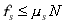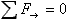f-sub-s is LESS THAN OR EQUAL TO mu-sub-s TIMES N

The force of static friction is less than or equal to the coefficient of static friction times the normal force.

Static friction only applies in the case of an object that is static (at rest). In the case of an object that is in equilibrium and is subject to a force of static friction, the force of static friction is whatever it has be in order to keep the object in equilibrium. In such a case, the force of static friction is typically determined by means of the equilibrium condition. But there is a limit to how much static friction force there can be. The limit is mu-sub-s times N. If the static friction force needed to keep an object in equilibrium is greater than mu-sub-s times N, then the object is not in equilibrium; it slips.

 Pitfall Avoidance Notes: 1. The only time the equals sign in the relation applies is when the object is on the verge of slipping. 2. mu-sub-s is not the frictional force. mu-sub-s is the coefficient of static friction. The value of mu-sub-s can only be a pure number, a value with no units. The force of static friction does depend on mu-sub-s, but it is never equal to mu-sub-s. It can't be. The quantity mu-sub-s does not even have units of force.